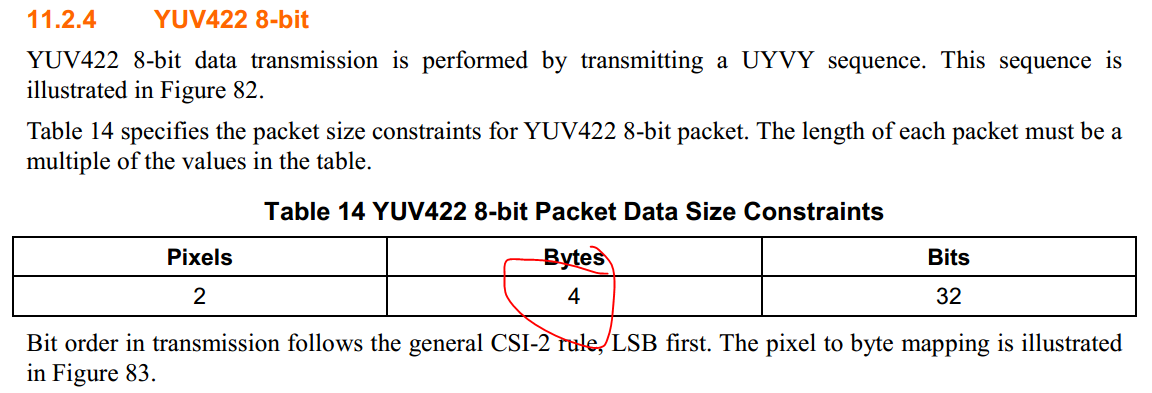• TI Thinks Resolved

# DS90UB954-Q1: YUV422 8bit block size

Part Number: DS90UB954-Q1

Hello,

How to define the block size? Take YUV422 8bit for example,  the below post describes the block size is 2,

e2e.ti.com/.../696737

but according to the MIPI CSI-2 spec, the packet size is 4byte, isn't 4 block size?In addition, the datasheet of DS90UB954 also describes the block size, I am confused about this,  for example, an RGB888 pattern would consist of 3-byte pixels and therefore require a 3-byte block size. A 2x12-bit pixel image would also require 3-byte block size, while a 3x12-bit pixel image would require nine bytes (two pixels) to send an integer number of bytes.   Could you please help to explain it how to define the block size?

Best regards

Kailyn

• Hello Kailyn,

For YUV422 8 bit, 2 pixels use 4 bytes, so 1 pixel uses 2 bytes which means the block size is 2. The goal is to ensure that the block size is such that each pixel uses an integer number of bytes. So in this case since each pixel uses 2 bytes, then the block size can be 2 which is already an integer.

Best Regards,

Casey

• In reply to Casey McCrea:

Hi Casey，

Thank you very much, that is to say, the block size is equal to the integer numbers of bytes with each pixel.

For example, YUV422 8bit is 2 pixel, 4bytes data, then one pixel is 2 bytes, so the block size is 2 instead of 4, isn't right?

The datasheet of DS90UB954 describes as this: The sizes are defined in the Mipi CSI-2 specification. For example, RGB888 requires a 3-byte block size which is the same as the pixel size. RAW10 requires a 5-byte block size which is equal to 4 pixels. RAW12 requires a 3-byte block size which is equal to 2 pixels.

Could you please help to explain why the block size is 3 of RGB888, and the block size is 5 of Raw10?

Best regards

Kailyn

• In reply to Kailyn Chen:

Hello Kailyn,

No, as I explained the block size is set such that each pixel uses an integer number of bytes, which means the pattern value for one block will not spread across multiple pixels.

For RGB888 there are 3 bytes per pixel. So 1/3 = 0.333 which is not a integer. With a block size of 3, then (1/3)*3 = 1 which is an integer

For YUV422 8 bit, there are 2 pixels for every 4 bytes, so 2/4 = 0.5 which is also not a integer. With a block size of 2, then (2/4)*2 = 1 which is an integer

For RAW10, there are 4 pixels for every 5 bytes, so 4/5 = 0.8, so multiply by 5 to get the nearest integer block size (4/5)*5 = 4 which is an integer

For RAW12, there are 2 pixels for every 3 bytes, so 2/3 = 0.666, so multiply by 3 to get an integer (2/3)*3 = 2 which is an integer

Best Regards,

Casey